Christmas

Exactly after 114 hours we sit down to Christmas Eve table. What day and what time it was when Dad said this sentence. The Christmas Eve table they sit down exactly on the 18-o'clock (6 PM).

Result

D =  21
h =  14

Solution:Leave us a comment of this math problem and its solution (i.e. if it is still somewhat unclear...):Be the first to comment!Next similar math problems:

1. How oldThe student who asked how many years he answered: "After 10 years I will be twice as old than as I was four years ago. How old is student?
2. Time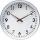16.2 days ..... how many hours is it?
3. Tram lines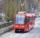Trams of five lines driven at intervals of 5,8,10,12 and 15 minutes. At 12 o'clock come out of the station at the same time. About how many hours again all meet? How many times have earch tram pass for this stop?
4. Bed timeTiffany was 5 years old; her week night bedtime grew by ¼ hour each year. If, at age 18, her curfew time is 11pm, what was her bed time when she was 5 years old?
5. Temperature increaseIf the temperature at 9:am is 50 degrees. What is the temperature at 5:00pm if the temperature increases 4 degrees Fahrenheit each hour?
6. The lowestThe lowest temperature on Thursday was 20°C. The lowest temperature on Saturday was 12°C. What was the difference between the lowest temperatures?
7. SchoolThere are 150 pupils in grade 5 . 2/3 of it are female. By what fractions are the males?
8. Change in temperatureStarting temperature is 21°C, the highest temperature is 32°C. What is the change in temperature?
9. Minutes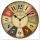Determine the difference in minutes: T1 = 2 3/20 h T2 = 2.3 h
10. Father and son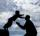Father is 44 years old, his son 16 years. Determine how many years ago was the father five times older than the son.
11. To thousands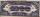Round to thousands following numbers:
12. Valid numberRound the 453874528 on 2 significant numbers.
13. RoundingThe following numbers round to the thousandth:
14. Flood waterFlood waters in some US village meant that the homes had to evacuate 364 people. 50 of them stayed at elementary schools, 59 them slept with their friends and others went to relatives. How many people have gone to relatives?
15. Round it0.728 round to units, tenths, hundredths.
16. Addition of Roman numbersAdded together and write as decimal number: LXVII + MLXIV
17. Rounding 2Round the following negative number: -143.021